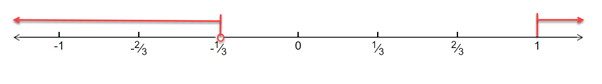# To solve the below inequality in terms of intervals and illustrate the solution set on the real number line - − 3 &lt; 1 x ≤ 1### Single Variable Calculus: Concepts...

4th Edition
James Stewart
Publisher: Cengage Learning
ISBN: 9781337687805### Single Variable Calculus: Concepts...

4th Edition
James Stewart
Publisher: Cengage Learning
ISBN: 9781337687805

#### Solutions

Chapter A, Problem 26E
To determine

## To solve the below inequality in terms of intervals and illustrate the solution set on the real number line -   −3<1x≤1

Expert Solution

The solution of the inequality is x<13 or x1 and the solution set on a real number line -### Explanation of Solution

Given: Inequality: 3<1x1

Formula Used:

An inequality compares two values, showing if one is less than, greater than, or simply not equal to another value.

Real number line is the line whose points are the real numbers.

Calculation:

Given: Inequality equation is 3<1x1

The above inequality can be broken down into:

3<1x and 1x1

Solving the first inequality, we have:

3<1x

Adding 3 to both the sides, we have:

3+3<1x+30<1x+30<1+3xxx1+3x>0

To solve the above inequality, we needto find the different intervals for which the inequality gives a value greater than 0 .

When x<13 :

x is negative and (1+3x) is negative

Thus, x1+3x>0

So, x<13 isone of the solutions.

When 13<x<0 :

x is negative and (1+3x) is positive

Thus, x1+3x<0

So, 13<x<0 is not one of the solutions.

When x>0 :

x is positiveand (1+3x) is positive

Thus, x1+3x>0

So, x>0 is one of the solutions.

Combining all the solutions, we have the solution set as:

x<13 or x>0

Solving the second inequality, we have:

1x1

Subtract 1 from both the sides, we have:

1x1111x101xx0x1x0

To solve the above inequality, we need to find the different intervals for which the inequality gives a value less than or equal to 0 .

When x<0 :

x is negative and (x1) is negative

Thus, x1x>0

So, x<0 is one of the solutions.

When x=0 :

x is zero and (x1) is negative

Thus, x1x is undefined

So, x=0 is not one of the solutions.

When 0<x<1 :

x is positive and (x1) is negative

Thus, x1x<0

So, 0<x<1 is not one of the solutions.

When x=1 :

x is positive and (x1) is zero

Thus, x1x>0

So, x=1 is one of the solutions.

When x>1 :

x is positive and (x1) is positive

Thus, x1x>0

So, x>1 is one of the solutions.

Combining all the solutions, we have the solution set as:

x<0 or x1

Now, combining solutions from both the inequalities, we have:

x<13 or x>0 and x<0 or x1

Thus, solution set is x<13 or x1

Drawing the above inequality on a real number line, we have:Conclusion:

Hence, the solution of the inequality is x<13 or x1 and the solution set on a real number line -### Have a homework question?

Subscribe to bartleby learn! Ask subject matter experts 30 homework questions each month. Plus, you’ll have access to millions of step-by-step textbook answers!